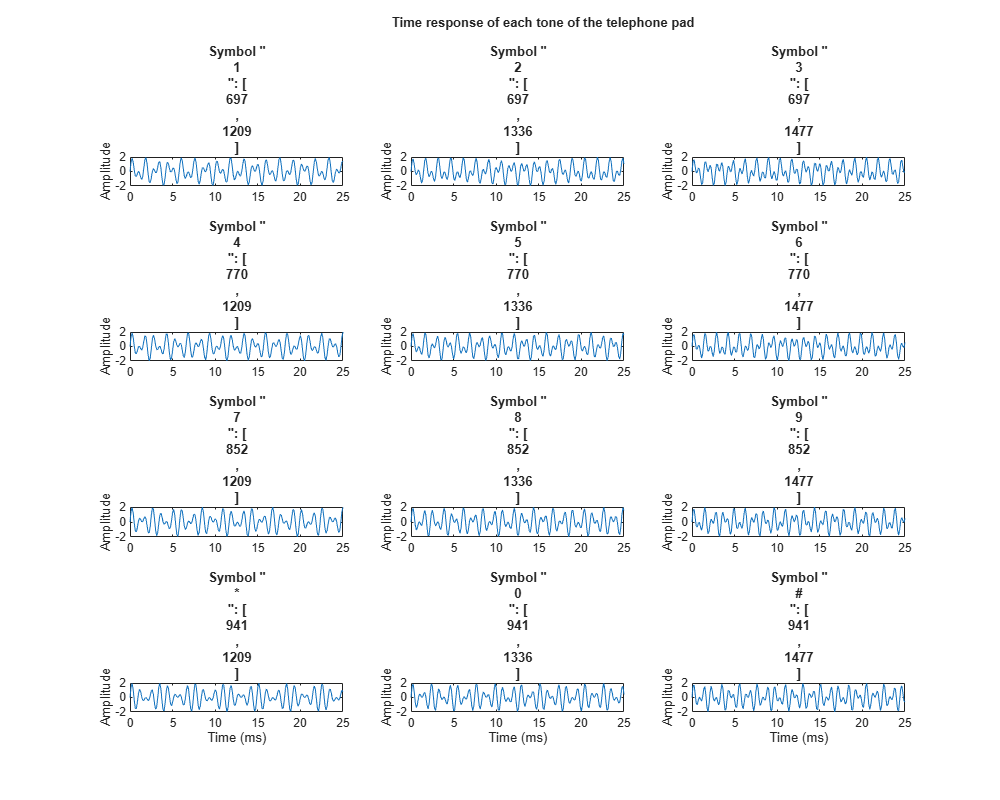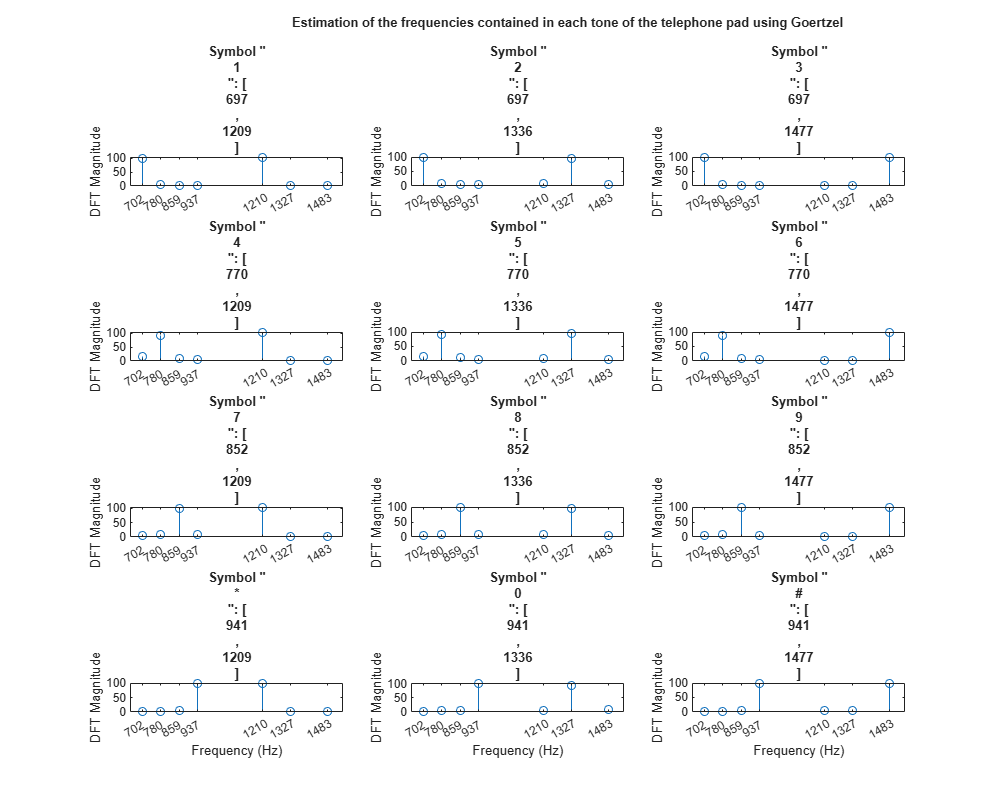Documentation

This is machine translation

Mouseover text to see original. Click the button below to return to the English version of the page.

DFT Estimation with the Goertzel Algorithm

This example shows how to use the Goertzel function to implement a DFT-based DTMF detection algorithm.

Dual-tone Multi-Frequency (DTMF) signaling is the basis for voice communications control and is widely used worldwide in modern telephony to dial numbers and configure switchboards. It is also used in systems such as in voice mail, electronic mail and telephone banking.

Generating DTMF Tones

A DTMF signal consists of the sum of two sinusoids - or tones - with frequencies taken from two mutually exclusive groups. These frequencies were chosen to prevent any harmonics from being incorrectly detected by the receiver as some other DTMF frequency. Each pair of tones contains one frequency of the low group (697 Hz, 770 Hz, 852 Hz, 941 Hz) and one frequency of the high group (1209 Hz, 1336 Hz, 1477Hz) and represents a unique symbol. The frequencies allocated to the push-buttons of the telephone pad are shown below:` `

Generate and plot a DTMF signal for each button on the telephone pad. Each signal has a sample rate of 8 Khz and a duration of 100ms.

```symbol = {'1','2','3','4','5','6','7','8','9','*','0','#'}; [tones, Fs, f, lfg, hfg] = helperDTMFToneGenerator(symbol, false); helperDFTEstimationPlot1(tones, symbol, Fs, f);```Playing DTMF Tones

Let's play the tones of phone number 508 647 7000 for example. Notice that the "0" symbol corresponds to the 11th tone.

```for i = [5 11 8 6 4 7 7 11 11 11] p = audioplayer(tones(:,i),Fs,16); play(p) pause(0.5) end```

Estimating DTMF Tones with the Goertzel Algorithm

The minimum duration of a DTMF signal defined by the ITU standard is 40 ms. Therefore, there are at most 0.04 x 8000 = 320 samples available for estimation and detection. The DTMF decoder needs to estimate the frequencies contained in these short signals.

One common approach to this estimation problem is to compute the Discrete-Time Fourier Transform (DFT) samples close to the seven fundamental tones. For a DFT-based solution, it has been shown that using 205 samples in the frequency domain minimizes the error between the original frequencies and the points at which the DFT is estimated.

```Nt = 205; original_f = [lfg(:);hfg(:)] % Original frequencies```
```original_f = 7×1 697 770 852 941 1209 1336 1477 ```
```k = round(original_f/Fs*Nt); % Indices of the DFT estim_f = round(k*Fs/Nt) % Frequencies at which the DFT is estimated```
```estim_f = 7×1 702 780 859 937 1210 1327 1483 ```

To minimize the error between the original frequencies and the points at which the DFT is estimated, we truncate the tones, keeping only 205 samples or 25.6 ms for further processing.

`tones = tones(1:205,:);`

At this point we could use the Fast Fourier Transform (FFT) algorithm to calculate the DFT. However, the popularity of the Goertzel algorithm in this context lies in the small number of points at which the DFT is estimated. In this case, the Goertzel algorithm is more efficient than the FFT algorithm.

```for toneChoice = 1:12 % Select tone tone = tones(:,toneChoice); % Estimate DFT using Goertzel ydft(:,toneChoice) = goertzel(tone,k+1); % Goertzel uses 1-based indexing end```

Plot Goertzel's DFT magnitude estimate of each tone on a grid corresponding to the telephone pad.

`helperDFTEstimationPlot2(ydft,symbol,f, estim_f);`Detecting DTMF Tones

The digital tone detection can be achieved by measuring the energy present at the seven frequencies estimated above. Each symbol can be separated by simply taking the component of maximum energy in the lower and upper frequency groups.

Appendix

The following helper functions are used in this example.